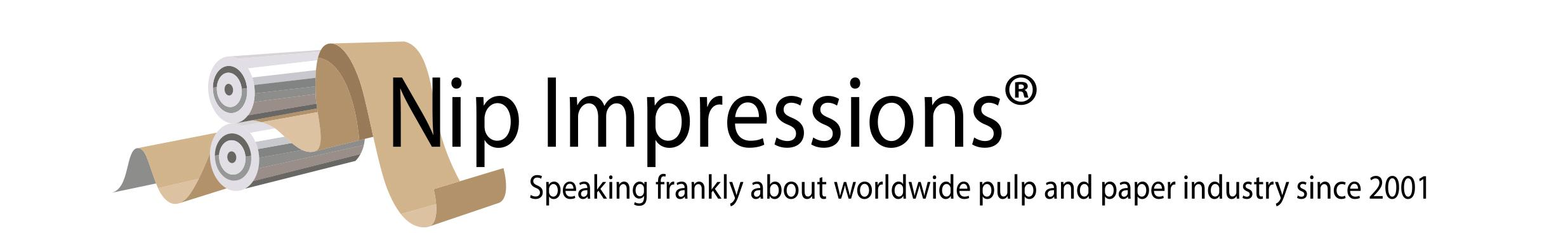Sun, Sep 24, 2023 12:11 Visitor

# Answer to this week's riddle

Again, the riddle:

Suppose you randomly placed a point on a number line.  What is the probability that the point is a rational number (that is a number expressed as one whole number divided by another)?

Answer: Zero! (in the limit, and assuming an infinitely accurate number line)Related Articles: